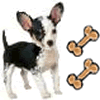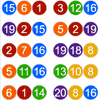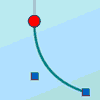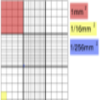Search by Topic

Resources tagged with Interactivities similar to A Roll of Patterned Paper:

Filter by: Content type:
Age range:
Challenge level:

There are 106 results

Broad Topics > Information and Communications Technology > InteractivitiesSquaring the Rectangle

Age 14 to 18 Challenge Level:

Can you find a way to turn a rectangle into a square?Attractive Tablecloths

Age 14 to 16 Challenge Level:

Charlie likes tablecloths that use as many colours as possible, but insists that his tablecloths have some symmetry. Can you work out how many colours he needs for different tablecloth designs?Rollin' Rollin' Rollin'

Age 11 to 14 Challenge Level:

Two circles of equal radius touch at P. One circle is fixed whilst the other moves, rolling without slipping, all the way round. How many times does the moving coin revolve before returning to P?Napoleon's Theorem

Age 14 to 18 Challenge Level:

Triangle ABC has equilateral triangles drawn on its edges. Points P, Q and R are the centres of the equilateral triangles. What can you prove about the triangle PQR?Excel Interactive Resource: Fraction Multiplication

Age 11 to 16 Challenge Level:

Use Excel to explore multiplication of fractions.Excel Interactive Resource: Long Multiplication

Age 11 to 16 Challenge Level:

Use an Excel spreadsheet to explore long multiplication.Overlap

Age 11 to 14 Challenge Level:

A red square and a blue square overlap so that the corner of the red square rests on the centre of the blue square. Show that, whatever the orientation of the red square, it covers a quarter of the. . . .Excel Interactive Resource: Interactive Division

Age 11 to 16 Challenge Level:

Use an Excel to investigate division. Explore the relationships between the process elements using an interactive spreadsheet.Cushion Ball Interactivity

Age 14 to 16 Challenge Level:

The interactive diagram has two labelled points, A and B. It is designed to be used with the problem "Cushion Ball"Ratio Pairs 3

Age 11 to 16 Challenge Level:

Match pairs of cards so that they have equivalent ratios.Factors and Multiples - Secondary Resources

Age 11 to 16 Challenge Level:

A collection of resources to support work on Factors and Multiples at Secondary level.Percentages for Key Stage 4

Age 14 to 16 Challenge Level:

A group of interactive resources to support work on percentages Key Stage 4.Excel Interactive Resource: Number Grid Functions

Age 11 to 16 Challenge Level:

Use Excel to investigate the effect of translations around a number grid.Excel Interactive Resource: the up and Down Game

Age 11 to 16 Challenge Level:

Use an interactive Excel spreadsheet to explore number in this exciting game!Excel Interactive Resource: Equivalent Fraction Bars

Age 11 to 16 Challenge Level:

A simple file for the Interactive whiteboard or PC screen, demonstrating equivalent fractions.Loci Resources

Age 14 to 16 Challenge Level:

This set of resources for teachers offers interactive environments to support work on loci at Key Stage 4.Excel Interactive Resource: Multiples Chain

Age 11 to 16 Challenge Level:

Use an interactive Excel spreadsheet to investigate factors and multiples.Excel Interactive Resource: Fraction Addition & Fraction Subtraction

Age 11 to 16 Challenge Level:

Use Excel to practise adding and subtracting fractions.Excel Interactive Resource: Haitch

Age 11 to 16 Challenge Level:

An Excel spreadsheet with an investigation.Solving with Euclid's Algorithm

Age 14 to 18 Challenge Level:

A java applet that takes you through the steps needed to solve a Diophantine equation of the form Px+Qy=1 using Euclid's algorithm.Red or Black Spinner

Age 3 to 16 Challenge Level:

A simple spinner that is equally likely to land on Red or Black. Useful if tossing a coin, dropping it, and rummaging about on the floor have lost their appeal. Needs a modern browser; if IE then at. . . .Countdown Fractions

Age 11 to 16 Challenge Level:

Here is a chance to play a fractions version of the classic Countdown Game.White Box

Age 7 to 18 Challenge Level:

This game challenges you to locate hidden triangles in The White Box by firing rays and observing where the rays exit the Box.Spinners Environment

Age 5 to 18 Challenge Level:

A tool for generating random integers.Fractions and Percentages Card Game

Age 11 to 16 Challenge Level:

Match the cards of the same value.Bow Tie

Age 11 to 14 Challenge Level:

Show how this pentagonal tile can be used to tile the plane and describe the transformations which map this pentagon to its images in the tiling.A Tilted Square

Age 14 to 16 Challenge Level:

The opposite vertices of a square have coordinates (a,b) and (c,d). What are the coordinates of the other vertices?Lottery Simulator

Age 7 to 16 Challenge Level:

Use this animation to experiment with lotteries. Choose how many balls to match, how many are in the carousel, and how many draws to make at once.3D Treasure Hunt

Age 14 to 18 Challenge Level:

Some treasure has been hidden in a three-dimensional grid! Can you work out a strategy to find it as efficiently as possible?Isosceles Triangles

Age 11 to 14 Challenge Level:

Draw some isosceles triangles with an area of $9$cm$^2$ and a vertex at (20,20). If all the vertices must have whole number coordinates, how many is it possible to draw?Treasure Hunt

Age 7 to 14 Challenge Level:

Can you find a reliable strategy for choosing coordinates that will locate the treasure in the minimum number of guesses?Square Coordinates

Age 11 to 14 Challenge Level:

A tilted square is a square with no horizontal sides. Can you devise a general instruction for the construction of a square when you are given just one of its sides?Polycircles

Age 14 to 16 Challenge Level:

Show that for any triangle it is always possible to construct 3 touching circles with centres at the vertices. Is it possible to construct touching circles centred at the vertices of any polygon?Graphical Interpretation

Age 14 to 16 Challenge Level:

This set of resources for teachers offers interactive environments to support work on graphical interpretation at Key Stage 4.Jam

Age 14 to 16 Challenge Level:

To avoid losing think of another very well known game where the patterns of play are similar.Investigating the Dilution Series

Age 14 to 16 Challenge Level:

Which dilutions can you make using only 10ml pipettes?Have You Got It?

Age 11 to 14 Challenge Level:

Can you explain the strategy for winning this game with any target?Proof Sorter - Quadratic Equation

Age 14 to 18 Challenge Level:

This is an interactivity in which you have to sort the steps in the completion of the square into the correct order to prove the formula for the solutions of quadratic equations.Sliding Puzzle

Age 11 to 16 Challenge Level:

The aim of the game is to slide the green square from the top right hand corner to the bottom left hand corner in the least number of moves.Using the Haemocytometer

Age 14 to 16 Challenge Level:

Practise your skills of proportional reasoning with this interactive haemocytometer.Rolling Around

Age 11 to 14 Challenge Level:

A circle rolls around the outside edge of a square so that its circumference always touches the edge of the square. Can you describe the locus of the centre of the circle?Estimating Angles

Age 7 to 14 Challenge Level:

How good are you at estimating angles?Countdown

Age 7 to 14 Challenge Level:

Here is a chance to play a version of the classic Countdown Game.Guesswork

Age 14 to 16 Challenge Level:

Ask a friend to choose a number between 1 and 63. By identifying which of the six cards contains the number they are thinking of it is easy to tell them what the number is.Poly Plug Rectangles

Age 5 to 14 Challenge Level:

The computer has made a rectangle and will tell you the number of spots it uses in total. Can you find out where the rectangle is?Konigsberg Plus

Age 11 to 14 Challenge Level:

Euler discussed whether or not it was possible to stroll around Koenigsberg crossing each of its seven bridges exactly once. Experiment with different numbers of islands and bridges.Partitioning Revisited

Age 11 to 14 Challenge Level:

We can show that (x + 1)² = x² + 2x + 1 by considering the area of an (x + 1) by (x + 1) square. Show in a similar way that (x + 2)² = x² + 4x + 4Tilted Squares

Age 11 to 14 Challenge Level:

It's easy to work out the areas of most squares that we meet, but what if they were tilted?Changing Places

Age 14 to 16 Challenge Level:

Place a red counter in the top left corner of a 4x4 array, which is covered by 14 other smaller counters, leaving a gap in the bottom right hand corner (HOME). What is the smallest number of moves. . . .Stars

Age 11 to 14 Challenge Level:

Can you find a relationship between the number of dots on the circle and the number of steps that will ensure that all points are hit?